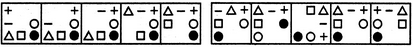# Non Verbal Reasoning - Series - Discussion

### Discussion :: Series - Section 1 (Q.No.3)

Each of the following questions consists of five figures marked A, B, C, D and E called the Problem Figures followed by five other figures marked 1, 2, 3, 4 and 5 called the Answer Figures. Select a figure from amongst the Answer Figures which will continue the same series as established by the five Problem Figures.

3.

Select a figure from amongst the Answer Figures which will continue the same series as established by the five Problem Figures.(A)     (B)     (C)     (D)     (E)       (1)     (2)     (3)     (4)     (5)

 [A]. 1 [B]. 2 [C]. 3 [D]. 4 [E]. 5

Explanation:

In each step, the CW-end element moves to the ACW-end position.

 Kranti said: (Jan 26, 2011) What is cw end & acw end positions ?

 Sundar said: (Jan 26, 2011) CW - clockwise ACW - anti-clockwise

 Ammu said: (Apr 11, 2011) Which is stating point in cw and which is the end point in cw ?

 Manimaran said: (Jun 14, 2011) Ya correct sundar.

 Almas Adhoni said: (Aug 7, 2011) What is the starting point and what is the ending point ?

 Hasini said: (Sep 9, 2011) Please explain this.

 Sandy said: (Nov 16, 2011) But option 1, 4 &5 are end same symbol in ACW how can you tell answer D.

 Ujwala said: (Nov 25, 2011) Please explain this. What is the starting point and what is the ending point ?

 Udaya Kumar said: (Dec 7, 2011) In analogy, the man proposes but the god disposes.

 Suresh said: (Aug 24, 2012) Please ! Explain. Which is the starting point ?

 Chethana said: (Aug 28, 2012) + is the cw corner in the option 'a'.after moving acw it goes to the left side.then nxt comes '-' in option 'b'.nw this has to move through acw.we can observe this move in option 'c'.in dis way the cycle cntinues.

 Abhi Mane said: (Mar 29, 2013) They moves in clockwise direction. After moving 1 symbol, select next symbol from anticlockwise direction.

 Pritam Chatterjee said: (Mar 28, 2014) In every block there is rotation in the clockwise direction. And in each block one symbol, I.E., the starting symbol is moving two places.

 Prathap said: (Apr 30, 2014) 1). Take A & B. Analyse them little, here + symbol moving from one end to another with two spaces. 2). Now look at C, In which - symbol which is behind "+" takes two spaces and joined with "+". 3). Similarly other symbols takes spaces. 4). In E, if bolded Dot takes two spaces, It will be like Answer "4" which is the answer.

 Suryani Mandal said: (Aug 30, 2016) I think the answer should be 1.

 Maharshi Dabhi said: (Nov 20, 2016) Last will come first and moves in anticlockwise.

 Pushpalatha said: (Jan 5, 2017) I feel the answer is 1 because if we are considering a clockwise movement then 1 figure is the right please check once.

 Eeka said: (May 20, 2017) I think 1 is the correct answer, because each symbol is jumping clockwise leaving 1 space. Will be understood easily if we put symbols in 3*3 table.

 Anjal said: (Nov 20, 2017) They moves in clockwise direction.

 Ashish said: (May 21, 2018) I think the answer should be 1 because each fig in the block is moving the same distance in CW fashion. So the answer should be 1.

 Asi said: (Oct 24, 2018) I am not understanding this. Please, anyone, help me to get it.

 Ravi Kumar said: (Nov 10, 2018) It is simple. Rotates cw direction. Cw is starts from + top of the left. In second position the + moves top right. Then - sign followed to +. After triangle followed to - sign. Then square followed to triangle. At finally the dot followed to square. So, 4th option is correct.